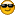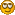###Author Topic: A small game - Let's solve 20 problems.  (Read 4154 times)

#### LaVanTien

• Guest##### A small game - Let's solve 20 problems.
« on: April 26, 2015, 03:15:35 pm »
Let's play a small game.
I hope that if you have time and have nothing to do to kill the time, then let solve these problems with me.
All greatest solution of each problems will be include in #1And no have end time.

If you are beginner (like me) and you can solve these 20 problems by yourself, you are really a genius (not like me, I can solve few of these), with a very bright future aheadAlthough these problems (except 19th problem) I wrote last week but some of them might still familiar with you, some are ordiginal, some are mix-up or remake of classic/legacy problems. And are designed just for stress rip of--------------------------------------
There are some rules you should consider to follow when post the solutions:
Quote
- Solutions must write in C language.
- Use just standard input and output, except 20th problem.
- Try to avoid including any third party library.
- You can post your codes here or paste onto an online paste website (like Pastebin or GitHub, etc...) and then post the link include your explainations here.

Enjoy the challenger...
("Italic" lines in these problems is made by accident not what I espect)
--------------------------------------
1. Write a program that prints "I like C language" to the screen without using "printf" function.
--------------------------------------
2. Write a program that enter two numbers x and y (0 <= x, y <= 10), print all expression make by these two numbers with errors handle.
Ex:

Quote
Input 1:
3 7

Output 1:
3 + 7 = 10
3 - 7 = -4
3 * 7 = 21
3 / 7 = 0
3 % 7 = 3
3 & 7 = 3
3 | 7 = 7
3 ^ 7 = 4
~ 3 = -4
~ 7 = -8
3 >> 7 = 0
3 << 7 = 384

Input 2:
4 0

Output 2:
4 + 0 = 4
4 - 0 = 4
4 * 0 = 0
Error: Division by zero.
Error: Division by zero.
4 & 0 = 0
4 | 0 = 4
3 ^ 0 = 4
~ 4 = -5
~ 0 = -1
4 >> 0 = 4
4 << 0 = 4

Input 3:
11 2

Output 3:
Error: Out of range.
--------------------------------------
3. Write a conversion program that convert pounds to kilograms, the program must not exit except when enter -1.
--------------------------------------
4. Write a program that take a list of string (the list not longer than 1000 members and length of members not longer than 80) except "end" and return another list of string but reversed.
Ex:

Quote
Input:
Pelles C
Hello World
end

Output:
C selleP
dlroW olleH
--------------------------------------
5. Write a program that take a binary number x (0 <= x <= 1111111111) and return a decimal number.
--------------------------------------
6. Write a program that calculate the value of this expression y = 2sqrt(x)/(1+x!)+x^20 (^ is expoment, x is integer and 0 <= x <= 20).
--------------------------------------
7. Write a program that count the number of prime numbers in any range [a, b] (0 <= a <= b <= 2000000000).
Ex:

Quote
Input:
0 10

Output:
4
--------------------------------------
8. Write a program that count each digits in any range [a, b] (0 <= a, b <= 2000000000).
Ex:

Quote
Input:
20 0

Output:
0: 3
1: 12
2: 3
3: 2
4: 2
5: 2
6: 2
7: 2
8: 2
9: 2
--------------------------------------
9. Write a program that take a string, delete a random character of the string, sort the string from smallest character to largest character, print out the sorted string.
Ex:

Quote
Input:
C is the best.

Output:
.Ceehisstt
--------------------------------------
10. Write a program that solve the cubic equation.
Ex:

Quote
Input 1:
1 2 -3 0

Output 1:
x1 = 1
x2 = -3
x3 = 0

Input 2:
1 2 3 0

Output 2:
x1 = -1 + 1.4142i
x2 = -1 - 1.4142i
x3 = 0
--------------------------------------
11. Write a program that find the largest and the second smallest number (in a list of integer numbers include n members) and their rate (0 < n <= 2000000000 and -2000000000 <= x <= 2000000000). Make sure your program use only one loop.
Ex:

Quote
Input:
5
1 -2 3 3 1

Output:
3 2
1 2
--------------------------------------
12. Write a program that take m, n and print out a sprial matrix size m*n (0 < m, n <= 20).
Ex:

Quote
Input:
4 4

Output:
1 2 3 4
12 13 14 5
11 16 15 6
10 9 8 7
--------------------------------------
13. Write a program that take 2 matrixes of same size n*n (0 < n <= 20), print out 2 invertible matrixes, sum of two given matrixes, product of two given matrixes.
Ex:

Quote
Input:
3

1 2 3
4 5 6
7 8 9

1 1 0
0 1 1
2 2 2

Output:
1 4 7
2 5 8
3 6 9

1 0 2
1 1 2
0 1 2

2 3 3
4 6 7
9 10 11

7 9 8
16 21 17
25 33 26
--------------------------------------
14. Write a program that find a largest subsequence of a integer sequence of n members (0 < n < 1000 and -1000 < x < 1000), the found subsequence must have smallest difference (Ex1: -4 and -2 so -4 is smallest difference, Ex2: 5 - 3 - 2 = 0 so 0 is called difference).
Ex:

Quote
Input:
5
1 2 0 4 -3

Output:
4
1 2 0 4
--------------------------------------
15. Write program to find sercurity risks. Give 4 character ('a'..'z') matrixes of same size n*n (represent of 4 servers), find positions where all 4 matrixes have the same member (represent sercurity risk).
Ex:

Quote
Input:
2

1 1
3 4

1 1
5 4

1 1
3 4

1 1
-2 4

Output:
1: (0, 0) (0, 1)
4: (1, 1)
--------------------------------------
16. Exactly 5 billions year from now, the Sun will eat the Earth. Write a program to find out how many nanoseconds from now til the day the Earth is eaten by the Sun. Do not pre-calculate and just print out the result, and do not use any kind of floating point number.
--------------------------------------
17. Write a program that take the number of disks (0 < n <= 100), count and print out the least steps needed to solve the "Towers of Hanoi" problem.
Detail about "Towers of Hanoi" problem: There will be 3 pillars (towers). You will be given a number of discs (frequently just few discs) put in the first pillar follow the order (The largest disc at bottom and the smallest disc on the top). Your task is move all the disc go to another pillars without messing the order (You cannot put the smaller disc bellow the larger one at each step).Ex:

Quote
Input:
5

Output:
31
--------------------------------------
18. Write a "Tic Tac Toe" game (play on console, each step enter a pair of integer number represent for coordinates of the game table 0..2 rows and 0..2 columns), a Player vs a Computer. Computer always go first, and your task is make the Computer smart enough to never be defeated.
--------------------------------------
19. "Next level" mines game (This problem was writen by Le Minh Hoang)
Give a mine field size m*n (1 <= m, n <= 200). Filled in by numbers (each number 0 <= x <= 8 ) represent for the number of mines around that cell (each cell have maximum 8 cells around it), each cell contain at most 1 mine. Write a program that determine exactly which cell has mine. 0 represent for "has mine" and 1 represent for "has not mine".
Ex:

Quote
Input:
4 4

1 3 3 1
2 3 4 4
3 6 5 3
1 3 3 3

Output:
1 0 0 1
0 1 1 0
0 0 1 1
1 1 1 0
--------------------------------------
20. Write a "next level" calculator, that can take an "infix expression" or a "postfix expression" ("Reverse Polish notation") at command line arguments, print out "postfix expression" or "infix expression" of given expression and its result.

Quote
The program must support:
- Signed floating point number.
- Maths operators:
+++ '+', '-', 'x', '/',
+++ 'd' (intergral divide, ex: 5 d 2 = 2),
+++ 'm' (modulus, ex: 5 m 2 = 1),
+++ '^' (expoment, ex: 2 ^ 3 = 8 ),
+++ '_' (radical, ex: 8 _ 3 = 2),
+++ 'L' (logarithm, ex: 8 L 2 = 3),
+++ 'l' (Neper logarithm, ex: 8 l = 2.07944 (5 digits accuracy)),
+++ '!' (factorial, ex: 5 ! = 120),
+++ 'C' (combination, ex: 4 C 2 = 6),
+++ 'A' or 'P' (permutation, some countries take letter A, somes take P, ex: 4 A 2 = 12)
+++ "sin(" ')', "cos(" ')', "tan(" ')', "cot(" ')',
+++ "asin(" ')', "acos(" ')', "atan(" ')', "acot(" ')'.
- Apsolute: '|', '|'.
- Parathenes: '(', ')'.
- Math error handle (overflow, division by zero).
- Syntax error handle.
- Each token separated by one space.
- If you going to enter a "infix expression", put "i:" at begin, else, put "p:" at begin.
- The "r:" in output mean "result".

Ex:
Input 1:
i: 3 + 4 - 5 m 2

Output 1:
p: 3 4 + 5 2 m -
r: 6

Input 2:
p: 3 6 + l 2

Output 2:
Error: Syntax error.

Input 3:
i: 2 ^ sin( ( 8 / 3 ) l )

Output 3:
p: 2 8 3 / l sin ^
r: 1.01194
--------------------------------------
Appendix for beginner

Runtime calculate.
It's very simple... Hope this little technique will help you on your solving path.
Code: [Select]
`    #include<stdio.h>    #include<time.h>    // ...    int main(void) {        time_t begin, end;        double runtime;        begin = clock() // <-- Start the timer        // ... <-- Put all things you need to calculate runtime in here        end = clock() // <-- Stop the timer        runtime = (double)(end - begin) / CLOCKS_PER_SEC; // <-- Calculate run time        printf("%lf\n", runtime); // <-- Show the runtime of your program        // The runtime is accurate to microsecond        // If using "scanf" or "scanf_s" before then you should put these line for getchar() to work.        int c;        while((c = getchar()) != 10); // <-- clean the buffer        getchar(); // <-- Pause the screen til get hit, optional        return 0;    }`--------------------------------------
« Last Edit: October 14, 2015, 02:02:49 pm by LaVanTien »